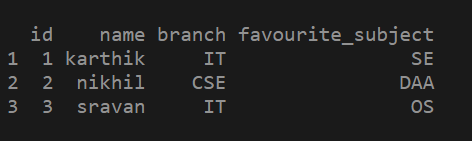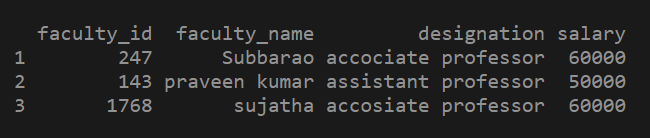Related Articles

# How to create a DataFrame from given vectors in R ?

• Last Updated : 02 Jul, 2021

In this article we will see how to create a Dataframe from four given vectors in R. To create a data frame in R using the vector, we must first have a series of vectors containing data. The data.frame() function is used to create a data frame from vector in R.

Syntax:

`data.frame(vectors)`

Example 1. Creating dataframe from given 4 vectors.

## R

 `# creating a vector with some value``id = ``c``(1, 2, 3)` `# creating another vector with some value``name = ``c``(``"karthik"` `, ``"nikhil"` `, ``"sravan"``)` `# creating another vector with some value``branch = ``c``(``"IT"` `, ``"CSE"` `, ``"IT"``)` `# creating another vector with some value.``favourite_subject = ``c``(``"SE"` `,``"DAA"` `, ``"OS"``)` `# passing the vectors into data.frame() function``# as parameters``df1=``data.frame``(id, name, branch, favourite_subject)` `# printing the data frame.``print``(df1)`

Output:Example 2:

## R

 `# creatingvvector 1 with some values``faculty_id = ``c``(247, 143, 01768)` `# creating a vector 2 with some values``faculty_name=``c``(``"Subbarao"``, ``"praveen kumar"``, ``"sujatha"``)` `# creating vector 3 with some values``designation=``c``(``"accociate professor"``, ``"assistant professor"``,``              ``"accosiate professor"``)` `# creating vector 4 with some data``salary = ``c``(60000, 50000, 60000)` `# passing the vectors to the data.frame() function``df3 = ``data.frame``(faculty_id, faculty_name, designation,salary)` `# printing the data frame created with 4 vectors``print``(df3)`

Output:My Personal Notes arrow_drop_up